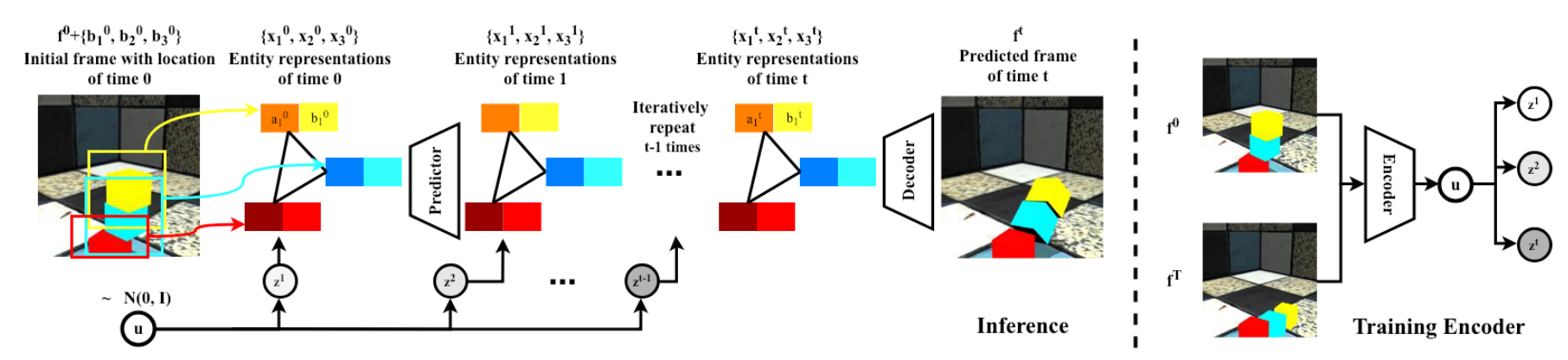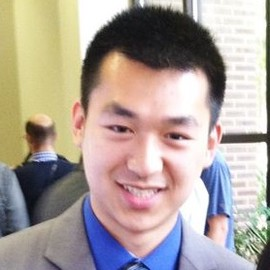# Compositional Video Prediction

Ye et al., 2019Source: Ye et al., 2019

## Summary

• Method for solving pixel-level future prediction given a single image
• Decompose scene into distinct entities that undergo motion and possibly interact
• Generates realistic predictions for stacked objects and human activities in gyms
• Links: [ website ] [ pdf ]

## Background

• Given a single image, humans can easily understand the scene and make predictions
• Many other works also model relationships between objects, but do some combination of:
• Use only simple visual stimuli
• Use state based input
• Rely on sequence of inputs
• Don’t make predictions in pixel space

## Methods

• Given starting frame $f^0$ and the location of $N$ entities $\{b^0_n\}^N_{n=1}$, predict $T$ future frames $f^1, f^2, \dots, f^T$
• Use entity predictor $\mathcal{P}$ for per-entity representations $\{x^t_n\}^N_{n=1}$
• $\{x^{t+1}_n\} \equiv \mathcal{P}(\{x^t_n\}, z_t)$
• $\{x^t_n\}^N_{n=1} \equiv \{(b^t_n, a^t_n)\}^N_{n=1}$
• $b^t_n$ is the predicted location
• $a^t_n$ is the predicted implicit features for entity appearance
• $a^0_n$ obtained using ResNet-18 on cropped region from $f^0$
• Interaction between entities modeled with graph neural network, edges are predetermined
• Use frame decoder $\mathcal{D}$ to infer pixels
• $f^t \equiv \mathcal{D}(\{x^t_n\},f^0)$
• Warp normalized spatial representation to image coordinates with predicted location for each entity
• Use soft masking for each entity to account for possible occlusions
• Add these masked features to features from initial frame $f^0$
• Single random latent variable $u$ to capture multi-modality of prediction task
• Yields per-timestep latent variables $z_t$, which are correlated accross time, via a learned LSTM
• Loss consists of three terms:
• Prediction: $l_1$ loss on decoded frame from predicted features and $l_2$ loss on predicted location
• Encoder: information bottleneck on latent variable distribution
• Decoder: $l_1$ loss on decoded frame from features extracted from the same frame (auto-encoding loss)

## Results

• Datasets:
• ShapeStacks: synthetic dataset of stacked objects that fall, different block shapes/colors and configurations
• Penn Action: real video dataset of people playing indoor/outdoor sports, annotated with joint locations
• Use gym activities subset: less camera motion, more similar backgrounds
• Metrics:
• Average MSE for entity locations
• Learned Perceptual Image Patch Similarity (LPIPS) for generated frames
• Due to stochasticity from random variable $u$, use best scores from 100 samples
• Baselines:
• No-Factor: only predicts at the level of frames
• No-Edge: no interaction among entities
• Pose Knows: also predicts poses as intermediate representation, predicts location but not appearance, no interactions
• Proposed method consistenly performs better than the simple baselines on ShapeStacks, but No-Edge is a fairly close second
• Adding adversarial loss, from Pose Knows, greatly improves performance of proposed method on Penn Action
• Qualitative results on Penn Action are generally not that convincing for any model

## Conclusion

• Relies on supervision of entity locations and graph structure
• Inclusion of latent variable to model multi-modality is interesting
• Better metrics for evaluating diversity and accuracy would be useful
• Poor performance on real world dataset suggests there is still a lot of room for improvement